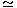# Materials and Components – Section 4

1.  Assertion (A): Diamond and carborudum are both very hard materials.

Reason (R): Valence band can be extremely strong.

 A. Both A and R are true and R is correct explanation of A B. Both A and R are true but R is not correct explanation of A C. A is true but R is false D. A is false but R is true

Explanation:

Valence bond results in hardness.

2.  The chemical properties of atoms are determined by all the electrons and their configuration.
 A. True B. False

Explanation:

Chemical properties are governed by valence electrons only.

3.  Nichrome is used for
 A. overload line wires B. heater coils C. lamp filaments D. all of the above

Explanation:

Because heater coil must be made of high resistivity material.

4.  If k is Boltzmann’s constant and T is absolute temp, then at room temp.
 A. kT0.025 eV B. kT0.5 eV C. kT1 eV D. kT100 eV

5.  If the valence electron is separated from a copper atom, the remaining part of atom has a net charge of
 A. 1 B. – 1 C. 0 D. + 3

6.  The dielectrics having only one kind of atoms are known as elemental dielectrics.
 A. True B. False

7.  For a core having a relative permeability μr, magnetic dipole moment per unit volume M and field strength H, the flux density B is
 A. B = μr (H + M) B. B = μ0 (H + M) C. B = μ0μr (H + M) D. B = μ0μr (H – M)

8.  The law that(where K is thermal conductivity, σ is electrical conductivity and T is absolute temperature) for all metals is equal to a universal constant is known as
 A. Lorentz law B. Wiedemann Franz law C. Curie Weiss law D. Kittel law

9.  In elemental dielectrics, the orientation and ionic polarization are absent.
 A. True B. False